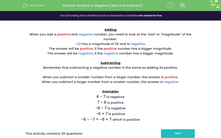# Identify Whether Addition and Subtraction Solutions Will Be Positive or Negative

In this worksheet, students will add and subtract positive and number answers to decide whether the answer is positive or negative.Key stage:  KS 3

Curriculum topic:   Number

Curriculum subtopic:   Use Four Operations for All Numbers

Difficulty level:#### Worksheet Overview

When you add a positive and negative number, you need to look at the 'size' or 'magnitude' of the number.

-32 has a magnitude of 32 and is negative.

The answer will be positive, if the positive number has a bigger magnitude.

The answer will be negative, if the negative number has a bigger magnitude.

Subtracting

Remember that subtracting a negative number is the same as adding its positive.

When you subtract a smaller number from a larger number, the answer is positive.

When you subtract a larger number from a smaller number, the answer is negative.

Examples

6 - 7 is negative

7 - 6 is positive

-6 - 7 is negative

-6 + 7 is positive

-6 - -7 = -6 + 7 which is positive

That's lots to take in, so you might like to look back to this introduction again while answering the questions. To do this, just click on the red button on the right of the screen.

### What is EdPlace?

We're your National Curriculum aligned online education content provider helping each child succeed in English, maths and science from year 1 to GCSE. With an EdPlace account you’ll be able to track and measure progress, helping each child achieve their best. We build confidence and attainment by personalising each child’s learning at a level that suits them.

Get started